# TimeMoney WorldTime, Money and Growth

HOMECOMPOUNDINGBORROWINGSAVING

# Capital Growth

### The Effect of Different Rates of Growth (Interest)

Here you can see how 1,000.00 (any currency) grows over a period of 5 years at different yearly interest rates, if the yearly interest is added to the capital at the end of each year, as it would be in an interest bearing bank deposit account.

The computations and graphs below are equally valid for a case where 1,000.00 is invested in a business yielding an annual profit as a percentage of the amount invested, and all the profit is re-invested at the end of the each year.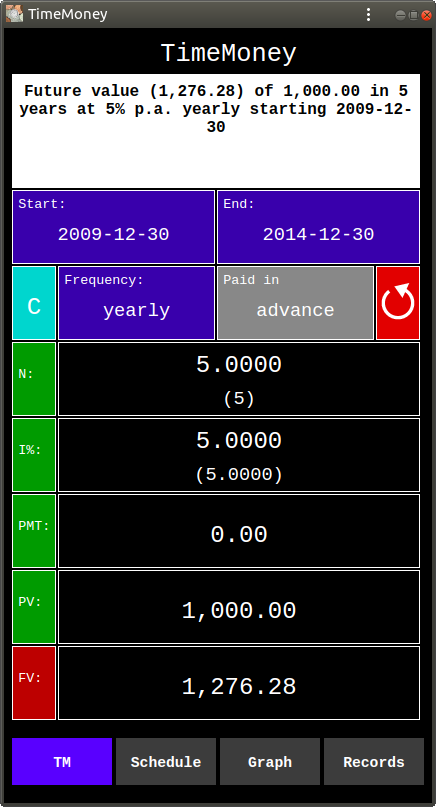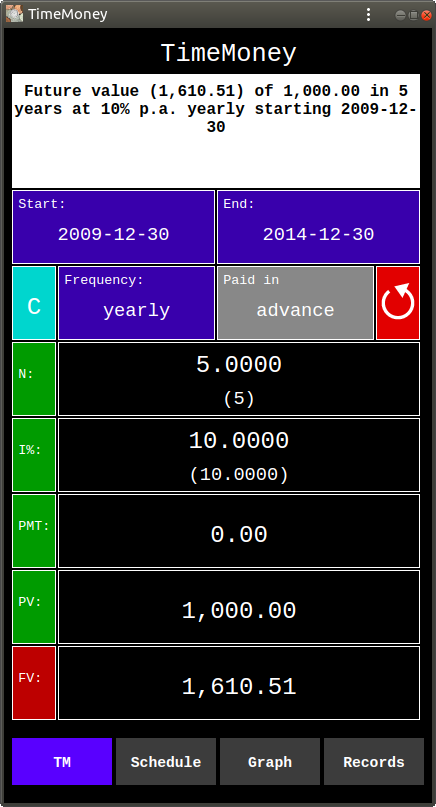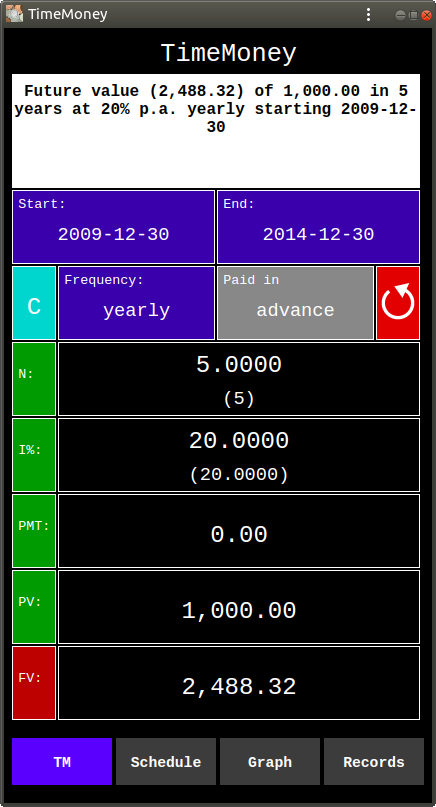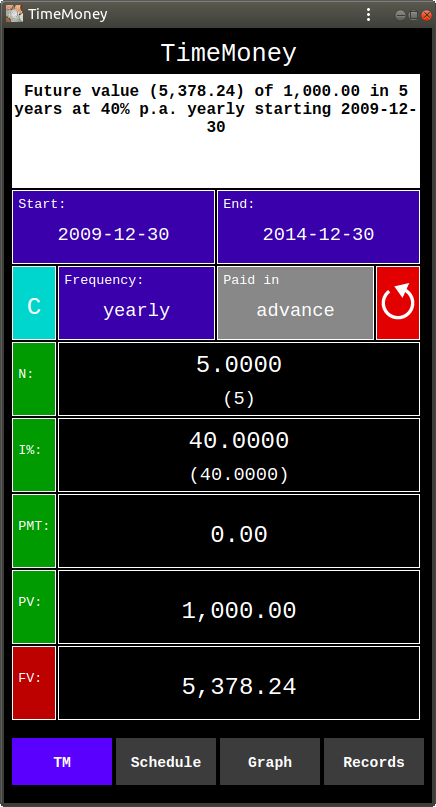Above are the computations of the Future Value (FV), that is of the Capital on 29 December 2014, to which the Present Value (PV) of 1,000.00, that is of the Capital on 30 December 2009, will grow after 5 years, at different annual (yearly) rates of growth (i%): 5%, 10%, 20%, 40%.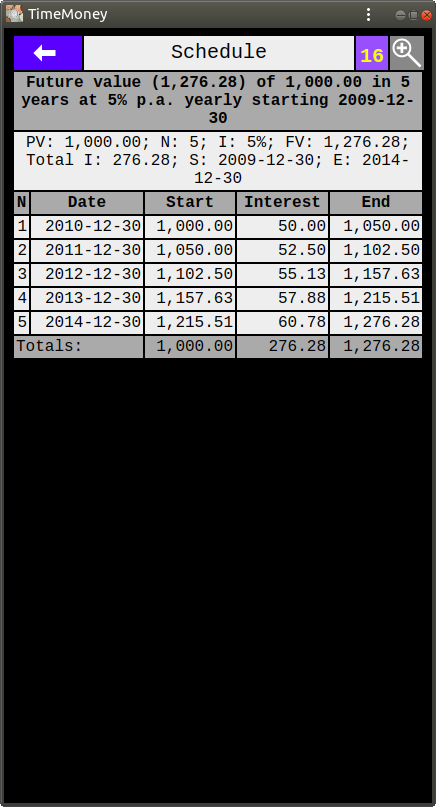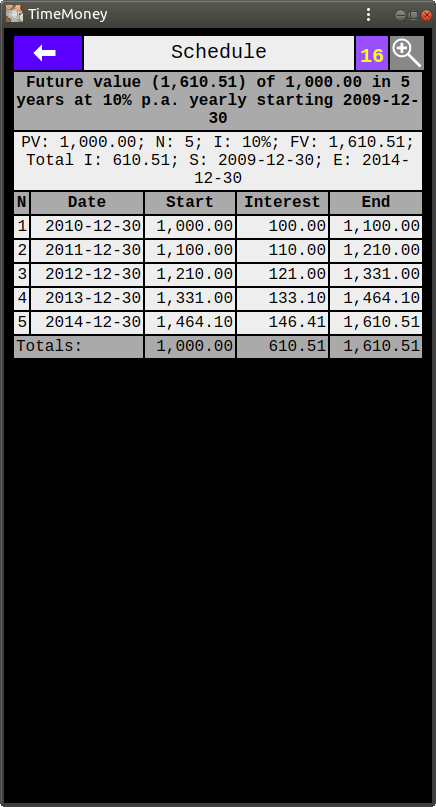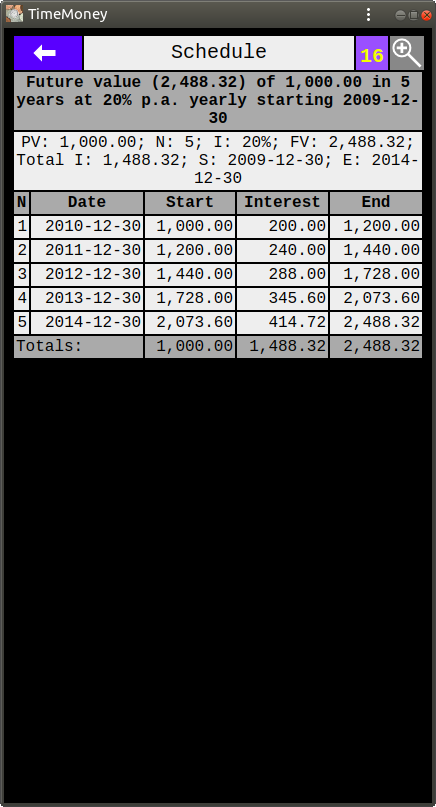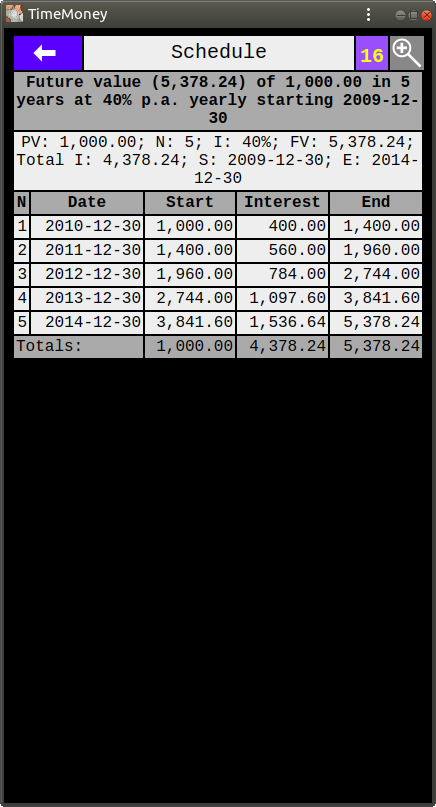Above are the capital accumulation schedules of the above computations.

The schedules show the growth of the capital within each of the 5 years (compounding periods).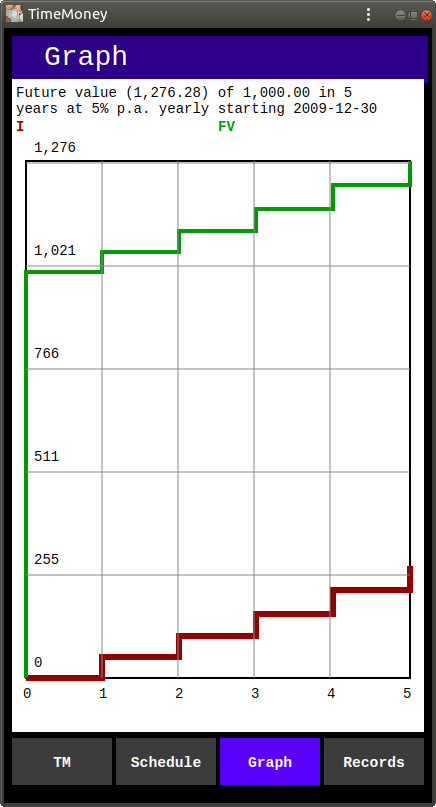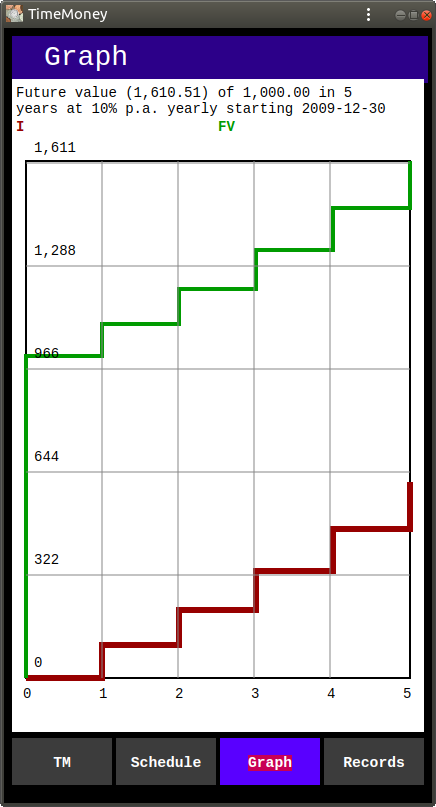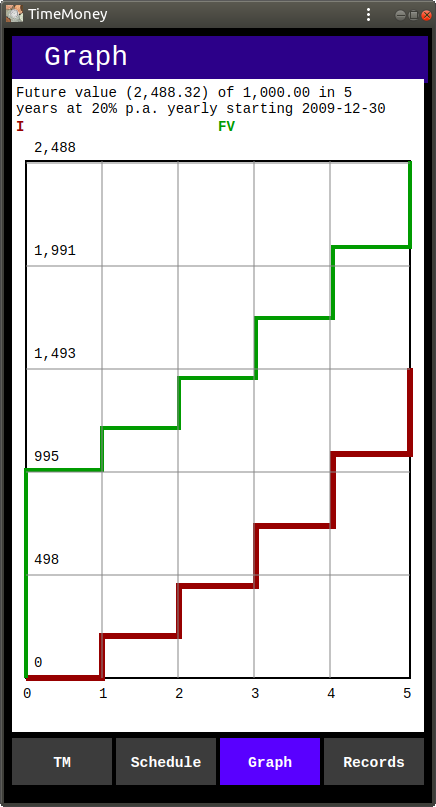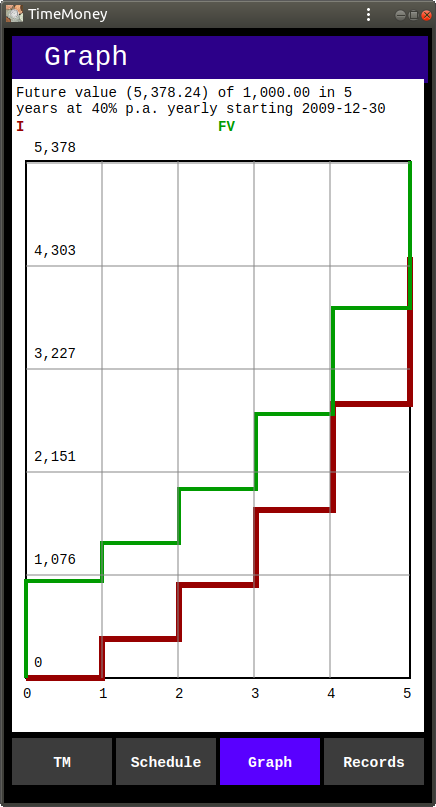Above are the graphs of the above computations.

The graphs show the growth of the capital within each of the 5 years (compounding periods).

The green line in the graphs is the Total Capital. The red line is the Interest (or profit) added at the end of each period.

Looking at the graphs side by side, one can see that with the increase of the growth rate the capital grows much faster with each successive period. At 5% growth rate the curves are almost straight and the steps are almost equal. At 40% growth the difference between each successive step is noticeably greater with each successive period.

The Growth Rate between each of the cases is doubled, but the profit difference between case 1 and case 2 is 2.21 times, between case 2 and case 3, 2.44 times and between case 3 and case 4, 2.94 times.

The formula for Future Value (FV) is:

FV = PV * (1 + r) n

Where:

PV is Present Value,
r is the yearly interest, as a fraction (i / 100)
n is number of years.

The above computations, schedules, and graphs were performed using TimeMoney by Dalasoft Ltd.

TimeMoney app is a universal Progressive Web Application (PWA) which works on any device (phone, tablet, desktop) on any operating system from your browser, as long as it supports modern JavaScript. And, if you install it on your device, you will be able to start it by clicking its badge on your Home Screen, like any other app on your device.
TimeMoney app is FREE. It has no ads and does not collect any data.
To try it out, use this link: TimeMoney .